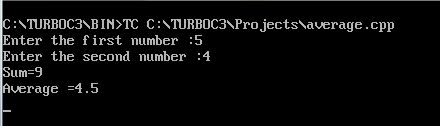UncleCoder.com

UncleCoder.com

Free programming examples and instructions

# CPP Program to find Average of two numbers

## CPP Program for how to find Average of two numbers

by Krishna

Posted on 27 Jun 2018 Category: c-plus-plus Views: 1189

CPP Program to find Average of two numbers

This section explains simple c++ program to read two numbers from the keyboard and display their average on the screen. Source code and output for the program is shown below.

``````#include<iostream>
#include<conio.h>
using namespace std;
int main()
{
float n1,n2,sum,average;
cout<”Enter the first number :”;
cout<”Enter the second number :”;
cin>>n2;
sum=n1+n2;
average=sum/2;
cout<<”Sum=”<<sum<<”\n”;
cout<<”Average =”<<average<<”\n”;
return 0;
getch();
}
``````

NOTE: some old versions of c++ use header  file <iostream.h>. so we should use iostream.h if the compiler does not support ANSI C++ features.

OUTPUT